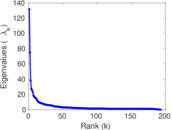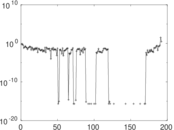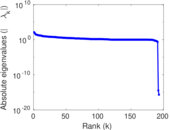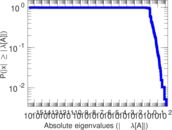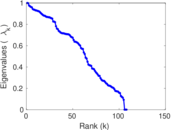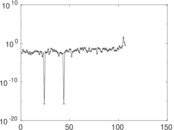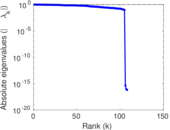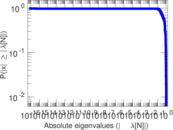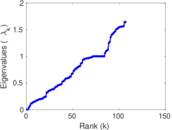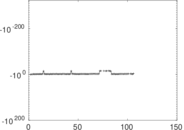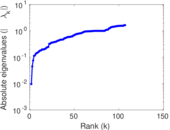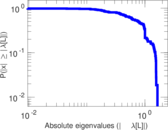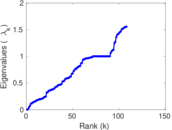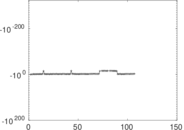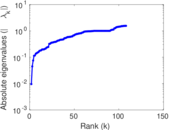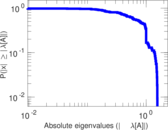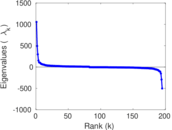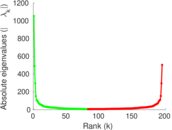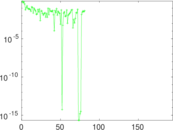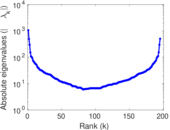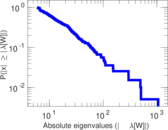# Wiktionary edits (tpi)

This is the bipartite edit network of the Tok Pisin Wiktionary. It contains users and pages from the Tok Pisin Wiktionary, connected by edit events. Each edge represents an edit. The dataset includes the timestamp of each edit.

 Code `mtpi` Internal name `edit-tpiwiktionary` Name Wiktionary edits (tpi) Data source http://dumps.wikimedia.org/ AvailabilityDataset is available for download Consistency checkDataset passed all tests Category Authorship network Dataset timestamp 2017-10-20 Node meaning User, article Edge meaning Edit Network formatBipartite, undirected Edge typeUnweighted, multiple edges Temporal dataEdges are annotated with timestamps

## Statistics

 Size n = 1,057 Left size n1 = 195 Right size n2 = 862 Volume m = 4,552 Unique edge count m̿ = 2,079 Wedge count s = 90,496 Claw count z = 3,787,800 Cross count x = 145,569,210 Square count q = 96,165 4-Tour count T4 = 1,135,766 Maximum degree dmax = 1,103 Maximum left degree d1max = 1,103 Maximum right degree d2max = 69 Average degree d = 8.613 06 Average left degree d1 = 23.343 6 Average right degree d2 = 5.280 74 Fill p = 0.012 368 4 Average edge multiplicity m̃ = 2.189 51 Size of LCC N = 746 Diameter δ = 14 50-Percentile effective diameter δ0.5 = 4.367 09 90-Percentile effective diameter δ0.9 = 7.991 18 Median distance δM = 5 Mean distance δm = 5.241 47 Gini coefficient G = 0.772 226 Balanced inequality ratio P = 0.186 841 Left balanced inequality ratio P1 = 0.123 682 Right balanced inequality ratio P2 = 0.214 411 Relative edge distribution entropy Her = 0.826 617 Power law exponent γ = 2.744 76 Tail power law exponent γt = 2.001 00 Tail power law exponent with p γ3 = 2.001 00 p-value p = 0.000 00 Left tail power law exponent with p γ3,1 = 1.671 00 Left p-value p1 = 0.564 000 Right tail power law exponent with p γ3,2 = 8.661 00 Right p-value p2 = 0.881 000 Degree assortativity ρ = +0.042 048 0 Degree assortativity p-value pρ = 0.055 248 5 Spectral norm α = 131.713 Algebraic connectivity a = 0.009 534 35 Spectral separation |λ1[A] / λ2[A]| = 1.754 40 Controllability C = 674 Relative controllability Cr = 0.638 258

## Plots

### Fruchterman–Reingold graph drawing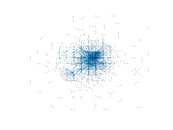### Degree distribution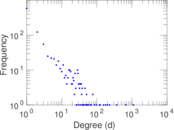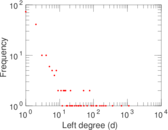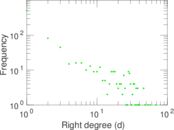### Cumulative degree distribution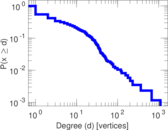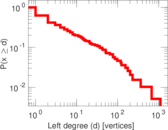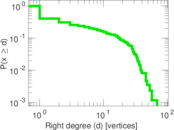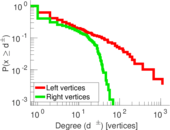### Lorenz curve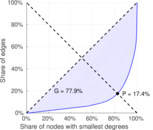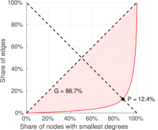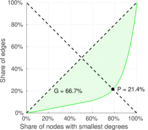### Spectral distribution of the adjacency matrix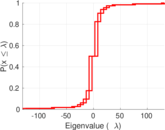### Spectral distribution of the normalized adjacency matrix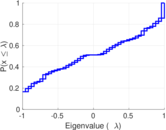### Spectral distribution of the Laplacian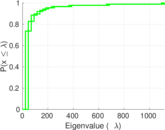### Spectral graph drawing based on the adjacency matrix### Spectral graph drawing based on the Laplacian### Spectral graph drawing based on the normalized adjacency matrix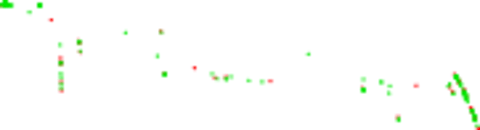### Degree assortativity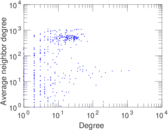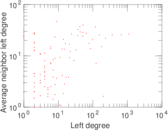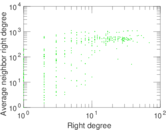### Zipf plot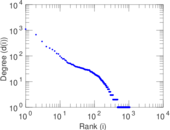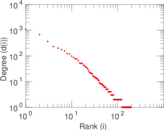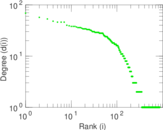### Hop distribution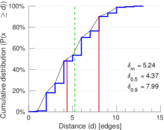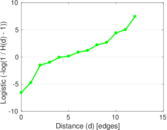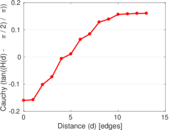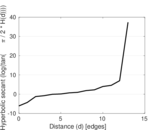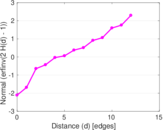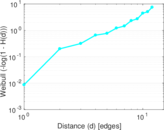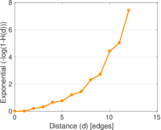### Double Laplacian graph drawing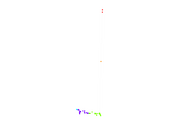### Delaunay graph drawing### Edge weight/multiplicity distribution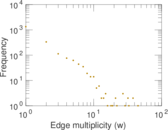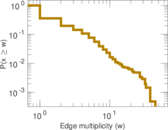### Temporal distribution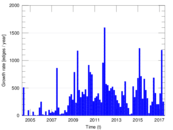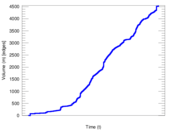### Temporal hop distribution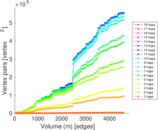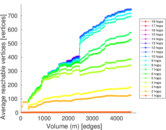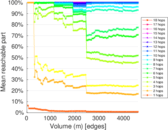### Diameter/density evolution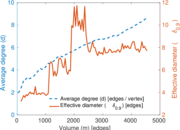### Matrix decompositions plots